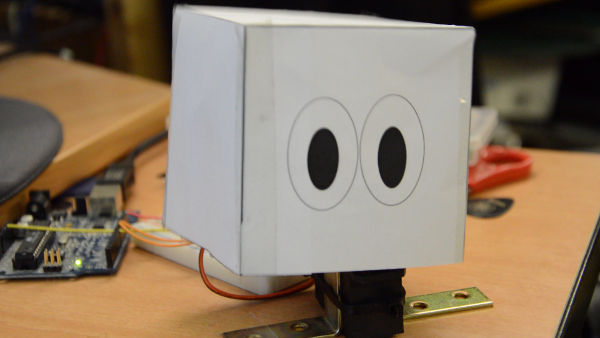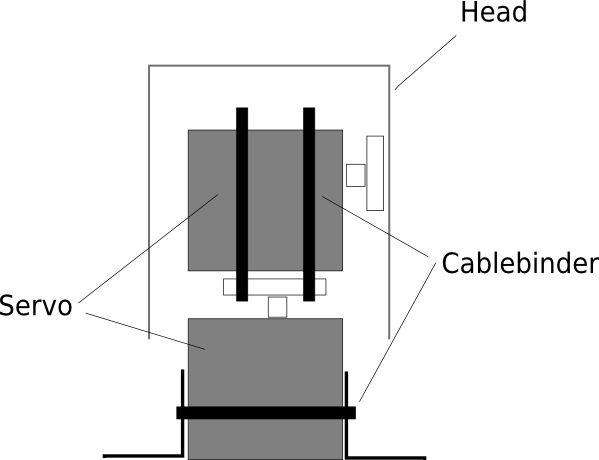## YesNoBot

This weekend I created the YesNoBot - a simple robot that is able to nod and shake its head.

I used two servo-motors a bunch of cablebinders, an arduino and a sheet of paper to create the bot. I wrote some build instruction after the videoThe servos get tied together using two cable binders as shown in the image below. I also used two brackets to stabilize the lower servo a bit. The head is cut from a sheet of paper and sticked to the upper servo using sticky tape.The servos get connected to 5+ and Gnd on the arduino, the control lines are connected to pin 9 and pin 10. Here is the code that runs on the arduino to move the head.

``````#include <Servo.h>

Servo s1;
Servo s2;

int p1 = 0;
int p2 = 0;

void setup() {

s1.attach(9);
s2.attach(10);
s1.write(90);
s2.write(22);
}

void loop() {
for (int i=0; i<2; i++) {
for( p1 = 45; p1 < 135; p1++) {
s1.write(p1);
delay(5);
}
for( p1 = 135; p1 > 45; p1--) {
s1.write(p1);
delay(5);
}

}
s1.write(90);

delay(30);
for ( int i=0; i<2; i++) {
for (p2 = 45; p2 > 0; p2--) {
s2.write(p2);
delay(15);
}
for (p2 = 0; p2 < 45; p2++) {
s2.write(p2);
delay(15);
}
}
s2.write(22);
delay(30);

}
``````